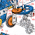## Wednesday, March 11, 2009

### How to linearize this function

I'm trying to solve an optimization problem via linear or mixed integer programming model. But, the objective function includes the following expression f(x)=1/x + 1/x^2 Please let me know how to formulate this function as linear or mixed integer programming model

There are several possibilities:

1. Solve using a piecewise linear formulation (e.g. using SOS2 variables).
2. Solve using as SLP (Successive LP). In this case for simplicity you may want to try just linearizing (Taylor approximation) the objective each cycle.
3. Use an NLP solver (e.g. MINOS, SNOPT or CONOPT). You could solve first as LP (eg by dropping this term) and use that solution as starting point.

1.Hey, cool job description: "math programming consultant".

I work using QLP in the hydropower field for operations and planning and have some unique control problems with nonlinear functions. I'm no wiz but seem to be able to make the clients happy...

One of our current problems is a sub-quadratic problem: the relationship is x^1.5 which we linearize inside the QLP. There are many other linearizations we've done though that we really need to fix it up afterwords with a nonlinear simulation - so we will likely be suboptimal but closer than any other speedy approach. Hopefully you can linearize and then fixup with a simulation?

cheers

2.Doh.

I realize now that your X^2 is in the objective function, not a constraint as I thought. If this really can't you just use a quadratic LP code? My LPs have objective functions with X*Y where both X and Y are calculated form decision variables - so I think it is kind of like your problem.

3.I assume the obj indicated is something like:

min/max y + 1/x + 1/x^2

4.